﻿ The Statistical Models Project (SMp) for Evaluations of Biological Radiation EffectsPublications are Open
Access in this journal
Article Versions
Export Article
• Normal Style
• MLA Style
• APA Style
• Chicago Style
Research Article
Open Access Peer-reviewed

### The Statistical Models Project (SMp) for Evaluations of Biological Radiation Effects

Terman Frometa-Castillo
American Journal of Applied Mathematics and Statistics. 2017, 5(4), 119-124. DOI: 10.12691/ajams-5-4-2
Published online: November 10, 2017

### Abstract

This document provides probabilistic-mechanistic models for describing the cell kill (K) and cell sub-lethal damage (SL) for one fraction with a dose of radiation that is absorbed by a living tissue; also this provides the K and SL formalisms for fractioned irradiation regimens. These models and formalisms are based on real mean behavior of cell survival (S) - a complement of K- and strong probabilistic-radiobiological foundations. The K and SL formalisms include all possible factors affecting the biological radiation effects: dose (d), fractionations (n), SL, and the temporal factors: cell repair and cell repopulation. It is discussed some aspects about the widely used linear-quadratic (LQ) S(d) model and LQ S(n,d) formalism, and one of its derivations, the BED (biologically effective dose). The SMp K(d) parameters can be obtained from S data, or using graphical/analytical tools developed by this study. These new formalisms will be useful for simulations of treatments, and together regional damage distribution for optimizations of the treatment planning.

### 1. Introduction

Until date the available data of radiosensitivity studies have been reported with the cell survival (S) in function of d, and mainly described by the linear-quadratic model, the LQ S(d) and its parameters α and β, while the fractioned irradiation regimens by the LQ S(n,d) formalisms or the biologically effective dose (BED). The S is a mixture of the undamaged and sub-lethally damaged cells, but there is not separate report of SL in function of d. Given S is a complement of K, then the current datasets reported with S can be done with K. and be used for determining the SMp K(d) parameters. Other alternative for obtaining these is through the known LQ S(d) parameters in fitted data characterized by a set of parameters α and β. As part of this work, a computational tool was developed for this purpose.

The use of LQ for describing the S function of dose is one of negative consequences that until date in the radiation oncology therapies it has not been used probabilistic functions for modeling stochastic processes and effects. The SMp has formulated pure probabilistic models enable of appropriately modelling these processes and effects.

The LQ S(n,d) formalism arose for characterizing S in fractioned irradiation regimens, and only has dependence of number of fractions (n) and dose (d). With the same purposes, the biologically effective dose (BED) was created. When researchers began to use the BED, they left to use directly the quantifications associated to fractions of survived cells affected by radiation, then they began to use a virtual and redundant radiobiological concept.

Recently the SMp has formulated in 1 the TCP/NTCP calculation methodologies that calculate the main radiobiological indices for radiotherapy, brachytherapy, and even radiation protection. These methodologies involve all possible elements for a quantitative evaluation: measurements, estimations and simulations, given the proposed methodologies establish two possible ways: 1- Using the phenomenological/mechanistic SMp TCP/NTCP models; and 2- Using computer-radiobiological simulators, which are not based on TCP/NTCP formulas, but their own probabilistic definitions employing criteria of the biological radiation effects (BRE). For determinations of BRE is essential the most acceptable K and SL formalisms, which is the main objective of this study.

We consider this study innovative because it proposes a probabilistic model for describing S or K, the SMp K(d) whose expression is less complex than the LQ S(d); besides based on probabilistic treatments, SL(d) model and the formalisms for K and SL were formulated. These formalisms define the BRE, and consider all factors affecting to this. In this study we make some discussions for some current radiobiological concepts related to the BRE.

### 2. The Cell Kill

2.1. The Liner-quadratic S(d), LQ S(d)

The current radiosensitivity studies have provided information of S, which has been modeled by the LQ S(d) as: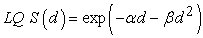(1)

where α and β: Parameters of this model

d: Dose.

The LQ S(d) is not wholly appropriate for describing the S because this and its parameters α and βare mathematical, and is a non-probabilistic model because its values are in the open interval. (0%; 100%), S=100% in d=0, while S=0% in d= positive infinity. These conditions are wholly out of reality, and are the same for whatever tissue T and radiation R. Nonetheless, the usefulness of this model is evident and demonstrated during many years, conditioned by it has some similarities with the probabilistic function. Ex. S continuously decreases when d increases, and takes values in the previous open interval.

2.2. The SMp K(d)

The SMp has classified in two types (P1 and P2) the behaviours of the mean values of the following stochastic processes/effects (SP/Es). P1: For values of the independent variable –In RT it is dose that is translated to absorbed energy- < a threshold value (TV) the process is 0%-deterministic, i.e. it will never occur. For values ≥ this threshold, the process is increased when the independent variable (IV) increases, from 0% to 100%, and is stochastic until a determined value of IV where the process becomes 100%-deterministic, i.e. it certainly occurs; and P2: For values of IV < a TV, the process is 0%-deterministic. For values ≥ this threshold, the process is increased when IV increases, from 0% to a maximum value, and later it begins to decrease until 0%, at this point the process is 0%-deterministic when IV increases.

Based on the SP/Es type P1, the SMp has formulated a new probabilistic-mechanistic K model: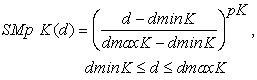(2)

where dminK: Threshold for cell kill

dmaxK: Minimum dose for a total cell kill

pK: Power of this model (pK>0)

d: Dose.

In d< dminK and d>dmaxK the cell kill is a respectively 0% and 100% deterministic effect.

The SMp K(d) or SMp S(d)=1-K(d) that is purely a probabilistic function, and for this reason it can be used as probabilistic-mechanistic model for describing the mean behavior of the cell kill. In this, K continuously increases when d increases, takes values in the closed interval 0%;100%, and defines clearly the stochastic and deterministic regions for each determined tissue T and radiation R in the radiosensitivity studies, because S=100% in d=dminK and S=0% in d=dmaxK.

The SMp K(d) parameters can be obtained from the S information, but when the data is not available, a set of parameters can be graphically and/or analytically derived from the equivalence between SMp S(d)model and LQ S(d) with α) and β . Ex. There is equivalence between LQ S(d, α=0.307 Gy-1, α/β=10 Gy) of 2 and SMp K(d, dminK=0.2 Gy, dmaxK=8 Gy, pK=0.4).

As part of this study a Matlab application was developed enable of manual and graphically determining the SMp K(d) parameters based on the equivalence between LQ and SMp models; varying the SMp parameters and taking into account the sum of absolute differences of both models, and that LQ model is not a probabilistic function, i.e. it does not clearly define the limits of stochastic and deterministic effects. This application is available through contacts with the authors.

### 3. The Cell Sub-lethal Damage (SL)

Based on the SP/Es type P2, the SMp has formulated a new probabilistic-mechanistic SL model: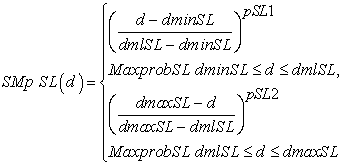(3)

where dminSL: Threshold for SL

dmlSL: Dose with the most likelihood of occurrence for SL

MaxprobSL: Maximum probability of SL

dmaxSL: Upper limit value for SL. dmaxSL=dmaxK

pSL1 and pSL2: Powers of this model (pSL1>0 and pSL2>0).

In d< dminSL and d>dmaxSL the cell sub-lethal damage is a 0%-deterministic effect. The values of SL(d) must be<= 1-(K(d)+U(d)) for all values of dose.

Nowadys the SMp K(d) parameters have an easy determinations from experiments, simulations or accpetable estimations, while for the SL only we have the choice of estimating their parameters because of the quoted radiosensibility studies only have reported the S in fucntion of dose, and it involves to undamaged cells (U) and SL.

### 4. The Linear Quadratic Cell Survival Formalism, the LQ S(n,d)

The LQ S(n,d) formalism was created for describing the S for fractioned irradiation regimens; but it is clearly seen this formalism only has dependence of the fractionations (n) and dose (d). i.e. it does not include all possible factors affecting BRE, like SL and temporal ones. For this reason, this formalism is incomplete.

This formalism is expressed as: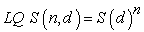(4)

using S(d) from Eq.(1):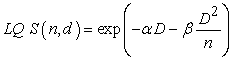(5)

where D=nd, and the parameters α and β are the same of the Eq. (1).

The quantifications of the LQ S(n,d) were replaced by BED, which is result of processing the exponential part of the Eq. (5), and written as: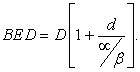(6)

The BED is a virtual and redundant radiobiological concept because of this is just a processed sub-part of the LQ S(n,d) formalism, its expression does not model neither physical nor biological quantity, is not associated to a real quantity.

When you create models for establishing the relationships among real quantities, you must not use them for creating new metrics, how has happened with the LQ S(n,d) formalism and the BED.

The LQ S(n,d) and its derivations, like the BED, are widely used in the conventional radiotherapy 3, 4, 5, 6, 7, 8, 9, 10, 11, and in the proton therapy 12, 13, 14.

The attempts for fitting mathematically S data have not done only with the LQ S(d), but with the linear-quadratic-cubic (LQC) by adding another cubic term to LQ model 15; and with a linear model in the high region (LQL model) for the high dose range proposed by 16. These modeling processes have mathematic foundations, and their parameters are purely mathematical.

The current linear-quadratic, LQ S(d) like our proposed SMp K(d) describes the S information just after the first fraction with a constant dose, other different analysis as 17 will be done using the SMp K formalism.

### 5. Irradiation to Living Tissues

The radiations in their interactions with living tissues can produce: K, SL and undamaged cells (U). These processes are stochastic, and it will be a better understanding of these processes, if these are renamed with probabilistic concepts, such as K with cell kill probability (CKP), SL with cell sub-lethal damage probability (CSLDP) and U with undamaged cells probability (UCP). The CKP will represent the mean quantity of cells that will be killed divided by total number of cells when same volumes of tissue are independently irradiated with a dose d in various experiments, while CSLDP and UCP will be associated respectively to sub-lethal damaged and undamaged cells.

As there are primary physical effects (photoelectric, Compton and formation of pairs) for photon interactions with the material, there are primary biological effects in the radiation interactions with a living tissue, such as K and SL.

Just after an irradiation to a living tissue with a constant dose d, in whatever j fraction, the following relationship is valid: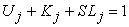(7)

where, Uj: Fraction of cells that have not been damaged until the jth fraction

Kj: Fraction of cells that have been killed until the jth fraction

SLj: Fraction of cells that have been sub-lethally damaged until the jth fraction.

Given the cell survival until the jth fraction (Sj) is a complement of Kj, then: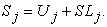(8)

The current reports of radiosensitivity studies use to S(d), which is a mixture of U(d) and SL(d), how is shown in the previous equation.

In an irradiated living tissue with n > 1, you must consider the SL and the temporal factors: the cell repair and cell repopulation, then after an inter-fraction time, for fractions j≥2 is valid, if it is assumed the cells die only by radiation: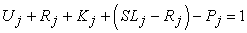(9)

where Uj: Fraction of cells that have not been damaged until the jth fraction

Rj: Fraction of cells that have been repaired until the jth fraction

Kj: Fraction of cells that have been killed until the jth fraction

SLj: Fraction of cells that have been sub-lethally damaged until the jth fraction

Pj: Ratio of repopulated cells and total number of cells until the jth fraction

Given: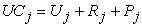(10)

here, UCj: Fraction of cells that are undamaged until the jth fraction; and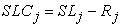(11)

where, SLCj: Fraction of cells that are sub-lethally damaged until the jth fraction.

Combining Eq. (9) and Eq. (10), then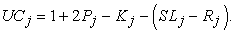(12)

### 6. The SMp K and SL Formalisms for Fractioned Irradiations

The treatments involving ionizing radiations are fractionated and performed with dose into the stochastic regions aimed to get the best possible efficacy of tumor control and the least possible complications in the normal tissues.

According to definition of K for n fractions with dose d, then: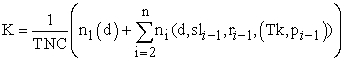(13)

where n: Number of fractions

TNC: Total number of cells

n1(d): Number of killed cells in the first fraction.

sli-1: Number of sub-lethal damaged cells before ith fraction.

ri-1: Number of repaired cells before ith fraction.

pi-1: Number of repopulated cells before ith fraction.

Tk: Time when the tumor repopulation begins.

ni(d): Number of killed cells in the fractions i≥2 that depends of d, sli-1, ri-1, Tk and pi-1.

Based on successive determinations of the K and SL expressions for n-fractions, and considering the Eq. (10) and Eq. (11), it was obtained the expressions from Eq. (14) to Eq. (19) represent the SMp K and SL formalisms for fractioned irradiations and function of: dose (d), number of fractions (n), cell sub-lethal damage (SL), cell repair (Rt), cell repopulation (Pt), and time when the tumor repopulation begins (Tk):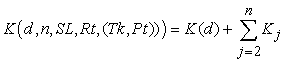(14)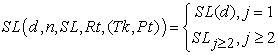(15)

where K(d): Cell kill for the first fraction with dose d

Kj: Cell kill for fractions further the first one

SL(d): Cell sub-lethal damage for the first fraction

SLj≥2(d): Cell sub-lethal damage for fractions further the first one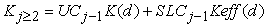(16)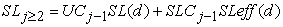(17)

where UCj-1: Fraction of cells that are undamaged before the jth fraction

SLCj-1: Fraction of cells that are sub-lethally damaged before the jth fraction

Keff(d): Effective K(d), which is related to contributions to K from SLCj-1.

SLeff(d): Effective SL(d), which is related to contributions to SL from SLCj-1.

Substituting the terms UCj-1 and SLCj-1according to their relationships of the Eq. (10) and Eq. (11), in the Eq. (16) and Eq. (17), then: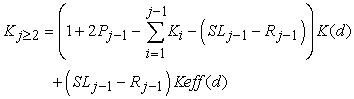(18)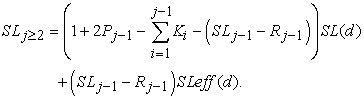(19)

There is a range of sub-lethal damages, for this reason in the formalisms for K and SL the effective K(d) and SL(d) (respectively Keff(d) and SLeff(d)) are used, which are related to contributions to K and SL from the sub-lethal damaged cells.

Given Keff(d) and SLeff(d) involve sub-lethal damaged cells: dminKe<dminK, dmaxKe<dmaxK, dminSLe<dminSL, dmlSLe<dmlSL, and dmaxSLe<dmaxSL. On the other hand, given Keff(d) and SLeff(d) are related to the same respective processes K(d) and SL(d), then the following assumptions will be considered: pKe=pK, p1SLe=p1SL, p2SLe=p2SL, and MaxprobSLe=MaxprobSL.

During a n-fractioned irradiation with dose d, the mean outcomes for K, SL and U function of the total absorbed dose (D=nd) shows how these are respectively associated to stochastic effects SMp type P1, P2 and P3, since from Eq. (7) you can see that in very low values of D, Uj=100%, and when Uj begins to decrease SLj gradually increases although some cells are repaired. For low values of D, Kj appears. At this point, Uj keeps its decrease, Kj slowly increases and SLj increases until a maximum value. Later as Kj increases due to this quantity is additive, and Uj decreases until 0%, SLj decreases until zero too.

For the new SMp formulations, this work is based on the following probabilistic-radiobiological foundations:

1. The SMp K(d) model is based on the well-known mean behavior of S in function of dose (d), the S is a complement of K; i.e. S(d)=1-K(d), and the similarities that the non-probabilistic LQ S(d) model has with the probabilistic one, in particular the SMp S(d). We have demonstrated how SMp S(d) is more appropriate than the LQ S(d) model for describing S.

2. The SMp SL(d) model is based on the general laws derived from a probabilistic analysis, Eq. (7) and Eq, (8), and the own definitions and characteristics of its radiobiological components, and we can demonstrate why SL is a stochastic effect SMp type P2. For example, taking the habitually used S, which is a mix of U and SL (S(d)=U(d)+SL(d)) you can define the two deterministic regions for SL=0%, where S=100% and U=100%; and S=0% and U=0%. Besides, it is logic if SL has two 0%-deterministic regions, then it has a maximum value into its stochastic region. Nowadays there is not experimental data concerning SL in function of doses (d), so its parameters should be estimated following the probabilistic and radiobiological constraints of this process. Ex. Radiobiologically the minimum dose for SL (dminSL) must be < minimum dose for K (dminK); and from a probabilistic point of view, the maximum probability for SL (MaxprobSL) must be <= 1-(K(d)+U(d)) evaluated in dose where SL(d) =MaxprobSL, the dmlSL (See the Eq. (7) and Eq. (8)). The SL(d) is maximum where K(d)+U(d) is minimum.

3. The SMp K and SL formalisms are based on the general laws derived from probabilistic analysis, like Eq. (9) – Eq. (12), as well as the Eq. (16) – Eq. (17) that represent the two possibilities for contributions to cell kill. In Eq. (18) the LQ S(n,d) formalism is a rare case for SLj-1=Rj-1 and Pj-1=0, because all cellular damages are not repairable nor the damaged cells are not repaired during inter-fraction time. The latter may be fulfilled for daily fractioned treatments, while the former is a condition very difficult to be satisfied, as well as the cell repopulation should not be omitted.

### 7. The Biological Radiation Effect

The quantifications of the radiation damage in living tissues can be done through K and SL that are direct biological effects of the radiation in its interactions with these tissues. It allows speaking quantitatively of the cumulative BRE, which is better than the cumulative of the BED, and better than the physical measure, the absorbed dose. The determinations of BRE will allow being used in the general expressions of the SMp TCP/NTCP models as independent variables instead dose variables, and in the computational-biological simulators that calculate TCP/NTCP without employing formulas of these radiobiological indices, but by using the own probabilistic definitions of these based on BRE criteria.

These formalisms will allow developing simulations for n fractions with better results than those obtained in 18, 19 where K and SL were used for only one fraction; as well as together the regional damage distribution for optimizations of the treatment planning how is described 20.

When a tissue volume V is irradiated with a non-uniform dose distribution and dose per fraction di in each fraction of volume vi, considering an uniform cell density in V, the K and SL for non-uniform dose distributions were obtained as: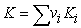(20)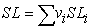(21)

where Ki: Fraction of cells that have been killed in vi

SLj: Fraction of cells that have been sub-lethally damaged in vi.

Although K and SL define BRE, the final evaluations of biological damages could be done through an equivalent K (Keq), where Keq ≡ BRE(K,SL).

### 8. Conclusion

Given our proposing SMp S(d) models is a probabilistic function (PF), and it has common elements with the LQ S(d), the SMp model will be applicable; and according to negative remarks pointed out to well-established, clinically validated, LQ S(d) model in clinical radiobiology, even the SMp S(d) could replace to this. Until date for fitting real datasets concerning radiosensitivity mathematical models have been used, in particular the LQ S(d) that has some similarities with the probabilistic models. All datasets, which have been fitted with models that have similarities with PFs can also be fitted with these.

The cell sub-lethal damage (SL) effect has been probabilistically characterized, which allowed obtaining a probabilistic model for this in function of the dose, the SMp SL(d). Given nowadays there is not separate experimental data concerning of this process, its model parameters should be estimated following the its probabilistic and radiobiological constraints.

The LQ S(n,d) formalism considers only as factors affecting biological radition effects (BRE), the dose(d) and the fractionation (n), so it is incomplete. All derivations from LQS(n,d) formalism, like biologically effective dose (BED), and the models using this formalism are incomplete too. This formalism is a rare case of the SMp K formalism.

The SMp K and SL formalisms that define to the BRE are complete becasuse they are fucntion of all factors affecting to this.

The SMp K(d) is based on real data of cell survival or cell kill; while the SL(d) model, the K and SL formalisms are based on strong probabilistic and radiobiological foundations.

We have provided some conclusions, such as: 1-The widely used BED is only a processed sub-part of the incomplete LQ S(n,d) formalism, and a virtual-redundant radiobiological concept; and 2- There is not BED, but BRE define by K and SL formalisms, so the BED must be replaced by the BRE, because of treating virtual and redundant concepts is anti-pedagogical.

### Acknowledgements

The most sincere acknowledgement to the Dr. Raul Piseaux-Aillon (Co-Founder of the SMp) for his appreciable contributions and advices.

### ReferencesThis work is licensed under a Creative Commons Attribution 4.0 International License. To view a copy of this license, visit http://creativecommons.org/licenses/by/4.0/